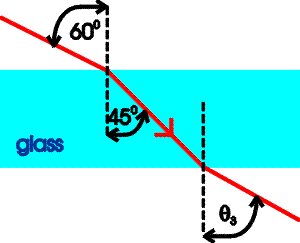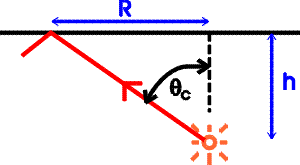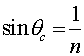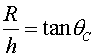Examples for refraction

Example #1Problem:

a.) A light beam enters a piece of glass from the vacuum at 60 degrees from the normal as shown and bend to 45 degrees from the normal once inside the glass. What is the index of refraction of the glass?

Solution:

Use Snell's law, n sin(45 degrees) = sin(60 degrees)

n = 1.22

b.) At what angle q3 does the light exit the slab of glass?

q3 = 60 o

Example #2Problem:

A diver carries a lamp below the surface of a still lake (index of refraction of water is 1.33) and circle of light is observed coming out of the surface with a radius of 16 m. How far is the diver below the surface?

Solution:

Light will not escape beyond the certain radius because the incident angle would exceed the critical angle. The critical angle is given by:The height is given byCombining these gives the depth h.

h = 14.1 m

Refraction's index